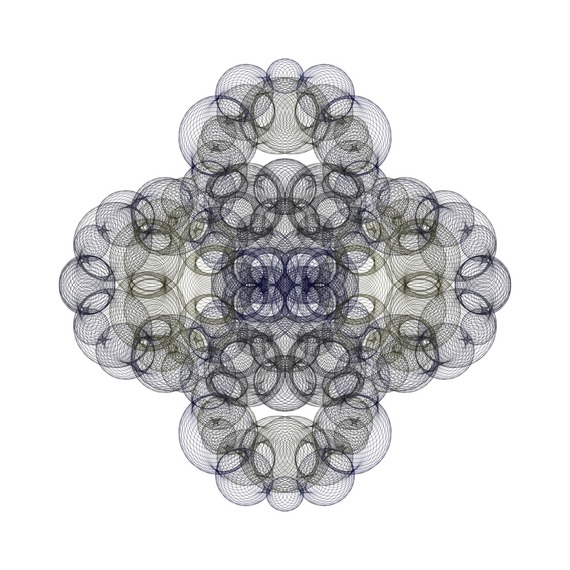# These Beautiful Images are Created by Drawing Circles

The circle is the most important closed curve in the world. Also, it is the most symmetrical shape in the plane. In addition to these facts, it is interesting that we can create an unlimited number of beautiful images by drawing circles. In order to create a beautiful symmetrical shape by this method, I draw a family of circles which are defined by some mathematical formulas. So, it is very similar to the method I mentioned in my previous post. But I think circles are better than line segments. I have created the following images by some computer programs. At the end of this post you can see the mathematical description of "4,000 Circles".

4,000 CirclesThis image shows 4,000 circles. For each k=1,2,3,...,4000 the center of the k-th circle is:

((2/3)(sin(6πk/4000))^3+(1/3)sin(26πk/4000), (2/3)(cos(6πk/4000))^3+(1/3)cos(26πk/4000))

and the radius of the k-th circle is:

(1/10)(sin(34πk/4000))^2+(1/12)(cos(136πk/4000))^4.